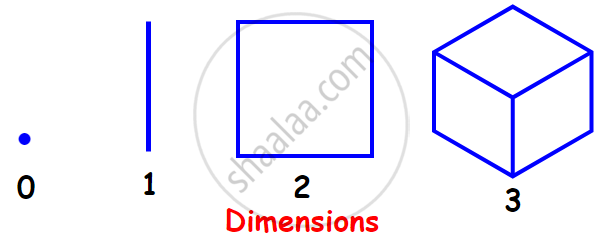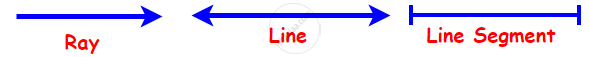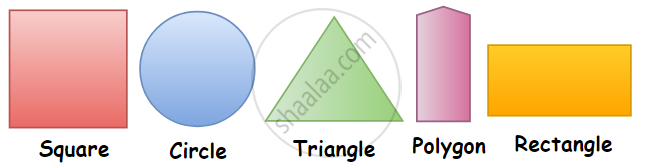# Concept for Basic Geometrical Ideas (2 -d)

## Notes

### Concept for Basic Geometrical Ideas:

The term ‘Geometry’ is the English equivalent of the Greek word ‘Geometron’. ‘Geo’ means Earth and ‘metron’ means Measurement.

#### What are Dimensions?

Dimensions in mathematics are the measure of the size or distance of an object or region or space in one direction. In simpler terms, it is the measurement of the length, width, and height of anything.

In our day to day life, we see several objects like books, balls, ice-cream cones, etc., around us which have different shapes.

Any object or space can be

One-dimensional (or 1D)

Two-dimensional (or 2D)

Three-dimensional (or 3D)

For example,#### 1. Zero Dimensional:

A point is a zero-dimensional object as it has no length, width, or height. It has no size. It tells about the location only. A point is dimensionless.#### 2. One Dimensional:

A line segment drawn on a surface is a one-dimensional object, as it has only length and no width.

For example,#### 3. Two Dimensional:

The 2-dimensional shapes or objects in geometry are flat plane figures that have two dimensions – length and width. Two-dimensional or 2-D shapes do not have any thickness and can be measured in only two faces.

A plane figure can be made of straight lines, curved lines, or both straight and curved lines. The circle, the square, the rectangle, the quadrilateral, and the triangle are examples of plane figures.If you would like to contribute notes or other learning material, please submit them using the button below.

### Shaalaa.com

Concept for Basic Geometrical Ideas [00:06:54]
S
0%